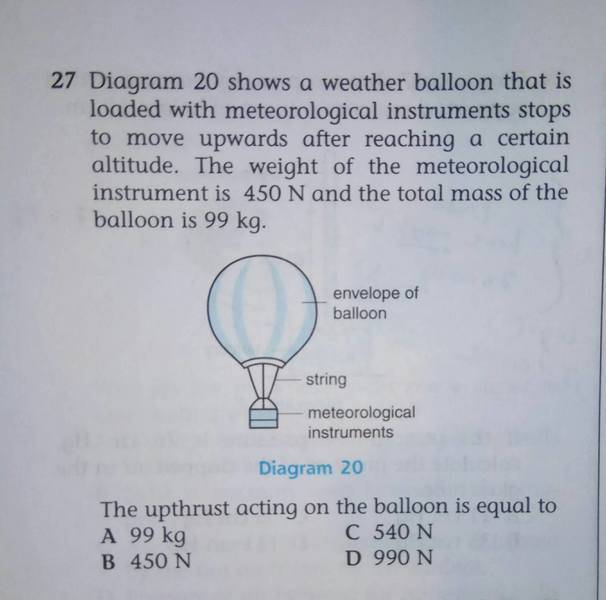# Archimedes' Principle and a weather balloon

## Homework Statement2. Homework Equations

Buoyant force= mg(mass of fluid displaced = Vpg (volume of fluid displaced)
Buoyant force=weight of object (if floating)

## The Attempt at a Solution

If the total mass is 99kg, then the total force acting downwards is 990N. It also said that the balloon "stops to move upwards after reaching a certain altitude" and from there I just assumed that it is floating, so upward force and weight are balanced.

My answer is D, but I'm not sure if it's correct because why did they give the weight of meteorological instrument?

•